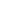# Alice and Bob's LazinessMubin_BUBT38CoU-BRACNet Inter Univers...7/11/47
Limits 2s, 512 MB

Father works for rail stations and his job is to supply and monitor equipment at the stations. The rail stations are connected with bidirectional railways, and each station can be reached from all other stations without forming any cycles. The equipment is measured by weight.

Father has to supply the equipment to the stations and also monitor the equipment weights frequently. He visits the stations in a manner where he checks all the station equipment from a starting station to a destination station. Sometimes, the equipment becomes unusable or problems occur, and the station master reports these issues. Father then replaces the equipment with new ones (not necessarily of the same weight) quickly.

Nowadays, Father is suffering from sickness, and he wants his two lazy sons, Alice and Bob, to take over his work. Despite their reluctance, Father divides the tasks between them. He assigns Alice to supply the equipment and Bob to monitor the equipment.

Alice and Bob are lazy, and they do not perform their tasks properly. When Father asks Alice to supply equipment to a station, Alice changes the weight of the equipment by its number of divisors.

When Father asks Bob to monitor equipment from station $U$ to station $V$, Bob only monitors those equipment weights that he hasn't monitored earlier during this specific visit from $U$ to $V$. He skips some stations' equipment and only checks stations further along the path from $U$ to $V$.

Father does not care about the equipment weight changed by Alice because it is easy to figure out. However, he wants to know how many stations' equipment Bob skipped each time Father asks Bob to monitor.

There are $N$ stations numbered from $1$ to $N$, connected by $N-1$ roads. One can reach every station from every other using the roads. Initially, Father gives Alice an array of weights $S$, and Alice is supposed to supply the equipment with weight $S_i$ to the $i$-th station for $1\leq i\leq N$.

There will also be $Q$ tasks. A task will be for Alice if it starts with $\texttt{A}$ or it will be for Bob if it starts with $\texttt{B}$.

For Alice's task, a station with the number $U$ and the equipment weight $W$ will be given. Alice is supposed to supply the equipment with weight $W$ to the station $U$.

For Bob's task, a starting station $U$ and a destination station $V$ will be given. Bob has to monitor the equipments along the simple path from $U$ to $V$.

Since Bob always skips the weight he did monitor earlier during that specific visit from $U$ to $V$, Father asks you to find the number of stations whose equipment Bob skipped.

## Input

The first line contains two integers $N$ and $Q$, representing the number of stations and the number of tasks, respectively.

The second line contains an array $S$ of $N$ integers, where $S_i$ indicates the initial equipment weight of the $i$-th station, which is supposed to be supplied by Alice initially.

The next $N-1$ lines describe the connections between stations. Each line contains two integers $U$ and $V$, indicating a connection between stations $U$ and $V$.

Finally, there will be $Q$ lines describing the tasks. Each task is either of the form $\texttt{A }U~W$ or $\texttt{B }U~V$.

Constraints

• $1 \leq N, Q, U, V \leq 2 \times 10^5$

• $1 \leq S_i, W \leq 10^6$

## Output

For each task of type $\texttt{B }U~V$, you have to print the number of stations that Bob skipped while visiting $U$ to $V$.

## Sample

InputOutput
8 7
10 12 16 20 15 26 25 7
1 2
3 1
4 8
1 4
2 5
6 7
2 6
B 5 7
B 6 3
B 7 8
A 4 24
B 7 8
A 2 21
B 5 7

1
1
2
1
2


After the changes of Alice, the initial $S$ will be $[4, 6, 5, 6, 4, 4, 3, 2]$.### Submit